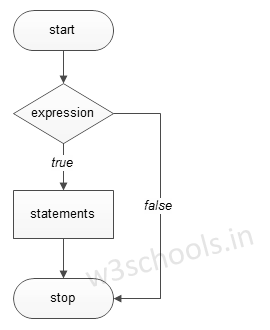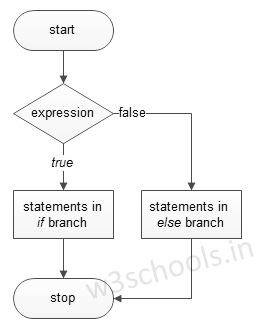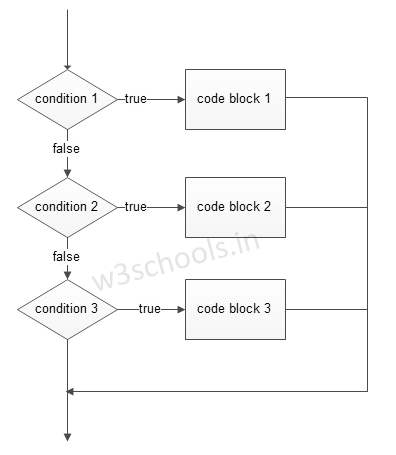# Python Decision Making

Decisions in a program are used when the program has conditional choices to execute a code block. Let's take an example of traffic lights, where different colors of lights lit up in different situations based on the conditions of the road or any specific rule.

It is the prediction of conditions that occur while executing a program to specify actions. Multiple expressions get evaluated with an outcome of either TRUE or FALSE. These are logical decisions, and Python also provides decision-making statements that to make decisions within a program for an application based on the user requirement.

Python provides various types of conditional statements:

Python Conditional Statements
StatementDescription
if StatementsIt consists of a Boolean expression which results are either TRUE or FALSE, followed by one or more statements.
if else StatementsIt also contains a Boolean expression. The if the statement is followed by an optional else statement & if the expression results in FALSE, then else statement gets executed. It is also called alternative execution in which there are two possibilities of the condition determined in which any one of them will get executed.
Nested StatementsWe can implement if statement and or if-else statement inside another if or if - else statement. Here more than one if conditions are applied & there can be more than one if within elif.

## if Statement

The decision-making structures can be recognized and understood using flowcharts.

Figure - If condition Flowchart:Syntax:
``````if expression:
``````
Example:
``````a = 15

if a > 10:
print("a is greater")
``````
Output:
`a is greater`

## if else Statements

Figure - If else condition Flowchart:Syntax:
``````if expression:
else:
``````
Example:
``````a = 15
b = 20

if a > b:
print("a is greater")
else:
print("b is greater")``````
Output:
`b is greater`

## elif Statements

elif - is a keyword used in Python replacement of else if to place another condition in the program. This is called chained conditional.

Figure - elif condition Flowchart:Syntax:
``````if expression:
elif expression:
else:
``````
Example:
``````a = 15
b = 15

if a > b:
print("a is greater")
elif a == b:
print("both are equal")
else:
print("b is greater")``````
Output:
`both are equal`

We can write if statements in both ways, within parenthesis or without parenthesis/ brackets, i.e. ( and ).

### Single Statement Condition

If the block of an executable statement of if - clause contains only a single line, programmers can write it on the same line as a header statement.

Example:
``````a = 15

if (a == 15): print("The value of a is 15")``````